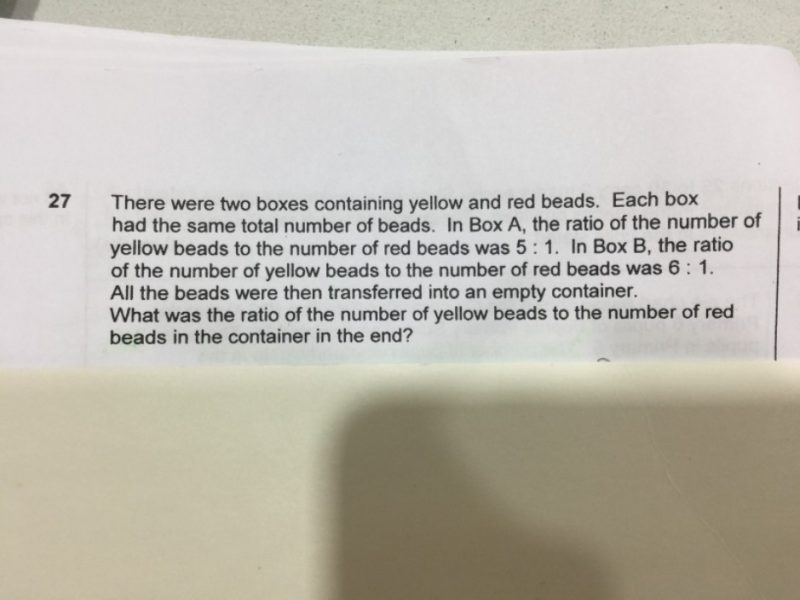# QuestionBox A: Ratio is 5 : 1

Total 6

Box B: Ratio is 6 : 1

Total is 7

Since the quantity in A and B is the same, find the LCM of 6 and 7, which is 42.

Box A, Y : R = 35 : 7

Box B, Y : R = 36 : 6

Total Y : R = 71 : 13

0 Replies 0 Likes